# Density (chemistry)

Density (symbol: ρ - Greek: rho) is a measure of mass per unit of volume. The higher an object's density, the higher its mass per volume. The density of an object equals its total mass divided by its total volume. An object made of a denser material (such as iron) will have less volume than an equal mass of some less dense substance (such as water). The SI unit of density is the kilogram per cubic metre (kg/m3):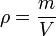$\rho = \frac{m}{V}$

where:

ρ is the object's density (measured in kilograms per cubic metre)
m is the object's total mass (measured in kilograms)
V is the object's total volume (measured in cubic metres)

Under specified conditions of temperature and pressure, the density of a fluid is defined as described above. However, the density of a solid material can be defined in several ways. Porous or granular materials have a density of the solid material, as well as a bulk density, which can be variable. For example, if you gently fill a container with sand, and divide the mass of sand by the container volume you get a value termed loose bulk density. If you took this same container and tapped on it repeatedly, allowing the sand to settle and pack together, and then calculate the results, you get a value termed tapped or packed bulk density. Tapped bulk density is always greater than or equal to loose bulk density. In both types of bulk density, some of the volume is taken up by the spaces between the grains of sand. The density of the sand grains, exclusive of the air between the grains, will be higher than the bulk density.

In candy making, density is affected by the melting and cooling processes. Loose granular sugar, like sand, contains a lot of air and is not tightly packed, but when it has melted and starts to boil, the sugar loses its granularity and some entrained air and becomes a fluid. When molded to make a smaller, compacted shape, the syrup tightens up and loses more air. As it cools, it contracts and gains moisture, making the already heavy candy even more dense.

## Other units

Density in terms of the SI base units is expressed in kilograms per cubic metre (kg/m3). Other acceptable SI units include grams per cubic centimetre (g/cm3), megagrams per cubic metre (Mg/m3) and kilograms per litre (kg/L).

In Imperial units or U.S. customary units, the units of density include pounds per cubic foot (lb/ft3), pounds per cubic yard (lb/yd3), pounds per cubic inch (lb/in3), ounces per cubic inch (oz/in3), pounds per gallon (lb/gal) for both the Imperial and the U.S. gallon, pounds per U.S. bushel (lb/bu), slugs per cubic foot (slugs/ft3), and others.

The maximum density of pure water at a pressure of one standard atmosphere is 999.861 kg/m3; this occurs at a temperature of about 3.98 °C (277.13 K).

From 1901 to 1964, a litre was defined as exactly the volume of 1 kg of water at maximum mass, and the maximum density of pure water was 1.000 000 kg/L (now 0.999 972 kg/L). However, while that definition of the litre was in effect, just as it is now, the maximum density of pure water was 0.999 972 g/cm3. During that period students had to learn the esoteric fact that a cubic centimetre and a millilitre were slightly different volumes, with 1 mL = 1.000 028 cm3 (often stated as 1.000 027 cm3 in earlier literature).

## Measurement of density

For a homogeneous solid object, the mass divided by the volume gives the density. The mass is normally measured with an appropriate weighing scale or balance and the volume may be measured directly (from the geometry of the object) or by the displacement of a liquid.

A very simple and commonly used instrument for directly measuring the density of a liquid is the hydrometer. A less common device for measuring fluid density is a pycnometer and a similar device for measuring the absolute density of a solid is a gas pycnometer.

Another instrument used to determine the density of a liquid or a gas is the digital density meter, based on the oscillating U-tube principle.

### Density of irregular shaped solid object heavier than water

One of the many statements attributed to the Archimedes principle is that an object immersed in a fluid apparently loses weight by an amount equal to the weight of the fluid displaced. That principle makes it possible to determine of the density of a solid object that is denser than water and is so irregular in shape that its volume cannot be measured directly.

First, the object's dry weight in air, Wd, is measured. Next, the weight of the object while submerged in water, Ws, is measured. Then the submerged object is removed from the water, the excess surface water is removed and the wet weight in air, Ww, is measured. The density of the object, ρo, is then obtained from:

(1)$\rho_o = \frac{m}{V} = \frac{W_d}{(W_w - W_s)/\rho_{water}} = \frac{W_d \cdot \rho_{water}}{W_w - W_s}$

and the volumetric porosity fraction, p can be obtained from:

(2)$p = \frac{W_w - W_d}{W_w - W_s}$

### Density of solid objects by x-ray diffraction crystallography

For some crystalline materials, density can be calculated using this equation which is based the molecular mass of the material and on information provided by x-ray crystallography:

(3)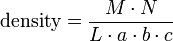$\mbox{density} = \frac{M \cdot N} {L \cdot a \cdot b \cdot c}$

where:

M is molecular mass
N is the number of ions, atoms or molecules in a unit cell
L is Loschmidt or Avogadro's constant
a, b, c are the lattice parameters

This equation holds only for crystal systems in which the angles between the crystal axes are ninety degrees, that is, for cubic, tetragonal, and orthorhombic systems. For crystals of lower symmetry the volume of the unit cell is no longer a × b × c, but must be generalized.

As an example of equation (3), the cubic lattice structure of crystalline sodium chloride (NaCl) is depicted in Figure 1. Each sodium ion is surrounded by six chloride ions and each chloride ion is surrounded by six sodium ions. The sodium chloride molecule, as such, has disappeared. This structural arrangement is in conformity with the x-ray reflection spectra of sodium chloride.

From a study of Figure 1, it can be deduced that each corner chloride ion is common to eight adjacent cubic cells so that only one-eighth of its mass contributes to the mass of the unit cell and thus all eight corner chloride units contribute the mass of one chloride ion. Likewise, the centered chloride ion on each face of the cubic cell is common to two adjacent cubic cells and thus all six of the face-centered chloride ions contribute the mass of three chloride ions. The total mass of the chlorine in the cell is therefore equal to the mass of four chloride ions.

Similarly, it can be deduced that each of the twelve sodium ions on the external faces of the cubic cell is common to four adjacent cubic cells so that only one-fourth of its mass contributes to the mass of the unit cell and thus the twelve external face sodium ions contribute the mass of three sodium ions. The one sodium ion in the center of the cell contributes all of it mass to the mass of the unit cell. The total mass of sodium in the cell is therefore equal to the mass of four sodium ions, and total mass of the cell may be taken as the mass of four molecules of sodium chloride.

The molecular mass of sodium chloride is 58.45 g/mol and the cube's lattice parameters a, b and c (height, width and length of the cell) are each 0.563 nm. Using Avogadro's constant of 6.022×1023 and equation (3) above:

density of NaCl = ( 58.45 × 4 ) ÷ ( 0.5633 × 6.022×1023 ) = 217.6 × 1023 g/nm3 = 2.176 g/cm3

That is within 0.5 percent of the 2.165 g/cm3 given as the density of NaCl in the Handbook of Chemistry and Physics.

### Density of gases

From the ideal gas law, the density of an ideal gas may be expressed as: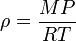$\rho = \frac {MP}{RT}$

and the density of a real gas (as differentiated from an ideal gas) may be expressed as:

(4)$\rho = \frac{MP}{ZRT}$

where Z is the compressibility factor, R is the molar gas constant, P is the pressure, M is the molecular mass, and T is the absolute temperature.

Thus, at an atmospheric pressure of 101.325 kPa, an absolute temperature of 273.15 K ( 0 °C ) and using R as 8.314472 m3·Pa·K-1·mol-1, the density of a real gas in kg/m3 is given by:

(5)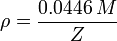$\rho =\frac {\mathrm{0.0446}\,M}{Z}$

At atmospheric pressure and 0 °C, most gases may be considered to be ideal and therefore the compressibility factor, Z, can be taken to be 1. At other conditions of temperature and pressure, where a gas may deviate from ideal gas behavior, the compressibility factor may be calculated using the van der Waals equation (see Compressibility factor (gases)#The van der Waals equation).

Whenever stating the density of a gas, it is important that the reference temperature and pressure (at which that density was obtained or calculated) be explicitly stated. Simply stating that the density is at standard conditions or at STP can lead to confusion since there is no universally accepted set of standard conditions (see Reference conditions of gas temperature and pressure).

## Density of various substances

Perhaps the highest density known is reached in neutron star matter (see neutronium). The singularity at the centre of a black hole, according to general relativity, does not have any volume, so its density is undefined.

Table 1: Densities of Various Substances
Substance Density
(kg/m³)
Substance Density
(kg/m³)
Iridium 22,650   Diamond 3,500
Osmium 22,610   Basalt 3,000
Platinum 21,450   Granite 2,700
Gold 19,300   Aluminum 2,700
Tungsten 19,250   Graphite 2,200
Uranium 19,050   Magnesium 1,740
Mercury 13,580   Polyvinylchloride (PVC) 1,300
Palladium 12,023   Seawater (15 °C) 1,025
Silver 10,490   Ice (0 °C) 917
Copper 8,960   Polyethylene 910
Iron 7,870   Ethyl alcohol 790
Steel 7,850   Gasoline 730
Tin 7,310   Liquid Hydrogen 68
Titanium 4,507   Aerogel 3

The densest naturally occurring substance on Earth is iridium, at about 22,650 kg/m3. Aerogel is the world's lightest solid. Also, note the low density of aluminum compared to most other metals. For this reason, aircraft are made of aluminum.

## The relation between temperature and the density of solids and fluids

The coefficient of cubic expansion, α, is the amount that a unit volume of liquid or solid increases for a temperature increase of 1 °C. Thus, if a volume of liquid or solid, V1, at temperature of T1 is raised in temperature to T2, then it has a new volume, V2, given by:$\mathrm{New \; volume} = \mathrm{Initial \; volume + Increase \; in \; volume}$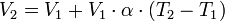$V_2 = V_1 + V_1 \cdot \alpha \cdot (T_2 - T_1)$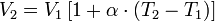$V_2 = V_1 \, [1 + \alpha \cdot (T_2 - T_1)]$

and therefore:

(6)$\frac {\rho_1}{\rho_2} = [1 + \alpha \cdot (T_2 - T_1)]$